Win up to 100% scholarship on Aakash BYJU'S JEE/NEET courses with ABNAT Win up to 100% scholarship on Aakash BYJU'S JEE/NEET courses with ABNAT

# JEE Main 2022 July 29 – Shift 2 Chemistry Question Paper with Solutions

The JEE Main 2022 July 29 – Shift 2 Chemistry Question Paper with Solutions is given on this page. Practising JEE Main 2022 question papers will be really beneficial for the students. It helps them to plan their studies by understanding the difficulty level of the exam. JEE Main 2022 answer keys can be used to find the error-free step-by-step solutions to the JEE Main 2022 exam. JEE Main 2022 question paper with solutions helps students to be familiar with the type of questions asked and thus be confident during the exam.

## JEE Main 2022 29th July Shift 2 Chemistry Question Paper and Solutions

SECTION – A

Multiple Choice Questions: This section contains 20 multiple choice questions. Each question has 4 choices (1), (2), (3) and (4), out of which ONLY ONE is correct.

1. Consider the reaction

$$\begin{array}{l} 4HNO_3(l)+3KCl(s)\rightarrow Cl_2 (g) + NOCl(g) + 2H_2O(g) + 3KNO_3 (s)\end{array}$$

The amount of HNO3 required to produce 110.0 g of KNO3 is

(Given : Atomic masses of H, O, N and K are 1, 16, 14 and 39 respectively.)

(A) 32.2 g

(B) 69.4 g

(C) 91.5 g

(D) 162.5 g

$$\begin{array}{l}4HNO_3\left(l\right) + 3KCl(s) \rightarrow Cl_2\left(g\right) + NOCl\left(g\right) + 2H_2O\left(g\right) + 3KNO_3\left(s\right)\end{array}$$
$$\begin{array}{l}\because 110 \text{ g of } KNO_3 \Rightarrow \text{ moles of } KNO_3 = \frac{110}{101}\\= 1.089~ \text{mol} \end{array}$$

As, 4 mole of HNO3 produces 3 mol of KNO3.

Hence, the moles of HNO3 required to produce 1.089 moles of KNO3 =

$$\begin{array}{l} = \frac{4}{3}\times 1.089 = 1.452 \text{ mol}\end{array}$$
$$\begin{array}{l}\text{Hence, mass of HNO3 required is}~ 1.452 \times 63\\ = 91.5 ~\text{g} (approx.)\end{array}$$

2. Given below are the quantum numbers for 4 electrons.

$$\begin{array}{l}A. n = 3, l = 2, m_l = 1, m_s = +1/2\\ B. n = 4, l = 1, m_l = 0, m_s = +1/2\\ C. n = 4, l = 2, m_l = –2, m_s = –1/2\\ D. n = 3, l = 1, m_l = –1, m_s = +1/2\end{array}$$

The correct order of increasing energy is

(A) D < B < A < C

(B) D < A < B < C

(C) B < D < A < C

(D) B < D < C < A

Energy of the sub-shell is given by, (n + l) rule.

 For (n + l) A 5 B 5 C 6 D 4

Hence, the correct order of increasing energy is

D < A < B < C

3.

$$\begin{array}{l}C(s) + O_2 (g)\rightarrow CO_2(g)+ 400 \text{ kJ}\end{array}$$

$$\begin{array}{l}C(s) + \frac{1}{2}O_2 (g)\rightarrow CO(g)+ 100 \text{ kJ}\end{array}$$

When coal of purity 60% is allowed to burn in presence of insufficient oxygen, 60% of carbon is converted into ‘CO’ and the remaining is converted into ‘CO2’. The heat generated when 0.6 kg of coal is burnt is _______.

(A) 1600 kJ

(B) 3200 kJ

(C) 4400 kJ

(D) 6600 kJ

Weight of coal = 0.6 kg = 600 gm

∴ 60% of it is carbon

$$\begin{array}{l} \text{So weight of carbon} =600 \times \frac{60}{100}=360 \text{ g}\end{array}$$
$$\begin{array}{l}\therefore \text{ moles of carbon } = \frac{360}{12}= 30 \text{ moles}\end{array}$$
$$\begin{array}{l}\underset{12~\text{moles}}{C}+ O_2 \longrightarrow CO_2\end{array}$$
$$\begin{array}{l}\underset{\underset{(60\% of\ total\ carbon)}{18 moles}}{C}+ \frac{1}{2}O_2 \longrightarrow CO\end{array}$$
$$\begin{array}{l}\therefore \text{Heat generated }= 12 \times 400 + 18 \times 100 = 6600~ \text{kJ}\end{array}$$

4. 200 mL of 0.01 M HCl is mixed with 400 mL of 0.01 M H2SO4. The pH of the mixture is ___.

[Given log 2 = 0.30, log 3 = 0. 48, log 5 = 0.70, log7 = 0.84, log 11 = 1.04.]

(A) 1.14

(B) 1.78

(C) 2.34

(D) 3.02

Molarity of resultant solution is given by

$$\begin{array}{l}\text{Molarity}= \frac{10}{600} \text{ of equivalents}\end{array}$$
$$\begin{array}{l}[H^+]=\frac{10}{600}\end{array}$$
$$\begin{array}{l}pH = -log\left[H^+\right]\end{array}$$
$$\begin{array}{l}pH = -log\left[\frac{10}{600}\right]=1.778\end{array}$$

5. Given below are the critical temperatures of some of the gases :

 Gas Critical temperature (K) He 5.2 CH4 190.0 CO2 304.2 NH3 405.5

The gas showing least adsorption on a definite amount of charcoal is

(A) He

(B) CH4

(C) CO2

(D) NH3

Extent of adsorption ∝ TC (critical temperature)

∵ Lower the TC, Lower will be the adsorption

Hence, Helium shows least adsorption on a definite amount of charcoal.

6. In liquation process used for tin (Sn), the metal

(A) is reacted with acid

(B) is dissolved in water

(C) is brought to molten form which is made to flow on a slope

(D) is fused with NaOH

Sol In liquation method, a low melting metal like tin can be made to flow on a sloping surface.

7. Given below are two statements.

Statement-I: Stannane is an example of a molecular hydride.

Statement-II: Stannane is a planar molecule

In the light of the above statement, choose the most appropriate answer from the options given below.

(A) Both Statement-I and Statement-II are true

(B) Both Statement-I and Statement-II are false

(C) Statement-I is true but Statement-II is false

(D) Statement-I is false but Statement-II is true

Sol Stannane or tin hydride is an inorganic compound with formula SnH4

Structure of SnH4 is∵ It is a non-planar molecule.

8. Portland cement contains ‘X’ to enhance the setting time. What is ‘X’?

$$\begin{array}{l}(\text{A})\ CaSO_4\cdot\frac{1}{2}H_2O\end{array}$$
$$\begin{array}{l}(\text{B})\ CaSO_4\cdot 2H_2O\end{array}$$
$$\begin{array}{l}(\text{C})CaSO_4\\(\text{D}) CaCO_3\end{array}$$

Sol Setting of cement: When mixed with water, the setting of cement takes place to give a hard mass.

This is due to the hydration of the molecule of the constituents and their rearrangement. The purpose of adding gypsum (CaSO4.2H2O) is only to slow down the process of setting of the cement so that it gets sufficiently hardened.

9. When borax is heated with CoO on a platinum loop, blue coloured bead formed is largely due to

$$\begin{array}{l}\left(A\right) B_2O_3 \\\left(B\right) Co\left(BO_2\right)_2\\ \left(C\right) CoB_4O_7\\ \left(D\right) Co\left[B_4O_5\left(OH\right)_4\right]\end{array}$$

Sol.

$$\begin{array}{l}Na_2B_4O_7 \xrightarrow{\ \ \Delta\ \ }2NaBO_2 + B_2O_3\end{array}$$
$$\begin{array}{l}B_2O_3 + CoO \rightarrow Co(BO_2)_2 \text{Cobalt metaborate(blue coloured)} \end{array}$$

10. Which of the following 3d-metal ion will give the lowest enthalpy of hydration (ΔhydH) when dissolved in water?

$$\begin{array}{l}\left(A\right) Cr^{2+} \left(B\right) Mn^{2+}\\ \left(C\right)Fe^{2+} \left(D\right) Co^{2+} \end{array}$$

Sol.

 Δhyd H (M+2) Cr – 1925 Mn – 1862 Fe – 1560 Co – 1640
$$\begin{array}{l}Mn^{2+}~ \text{has lowest }\Delta_{hyd}H\end{array}$$

11. Octahedral complexes of copper(II) undergo structural distortion (Jahn-Teller). Which one of the given copper(II) complexes will show the maximum structural distortion?

$$\begin{array}{l}\left(\text{en – ethylenediamine}; H_2N-CH_2-CH_2-NH_2\right)\end{array}$$
$$\begin{array}{l}\left(A\right) \left[Cu\left(H_2O\right)_6\right]SO_4\\ \left(B\right) \left[Cu\left(en\right)\left(H_2O\right)_4\right]SO_4\\ \left(C\right) cis-\left[Cu\left(en\right)_2Cl_2\right]\\ \left(D\right) trans-\left[Cu\left(en\right)_2Cl_2\right]\end{array}$$

Sol.

John teller distortion: Any non-linear compound remove its degeneracy to attain the stability.

Extent of John teller distortion depends upon metal ion as well as nature of ligand.

Stronger the ligand, more will be the John Teller distortion and more will be the stability.

Hence Trans [Cu(en)2Cl2] will exhibit maximum John Teller distortion.

12. Dinitrogen is a robust compound, but reacts at high altitudes to form oxides. The oxide of nitrogen that can damage plant leaves and retard photosyn-thesis is

$$\begin{array}{l}\left(A\right)NO\\\left(B\right)NO_3^-\\\left(C\right)NO_2\\ \left(D\right)NO_2^-\end{array}$$

Sol.

Higher concentration of NO2 damages the leaves of plant and retards photosynthesis.

13. Correct structure of γ-methylcyclohexane carbaldehyde is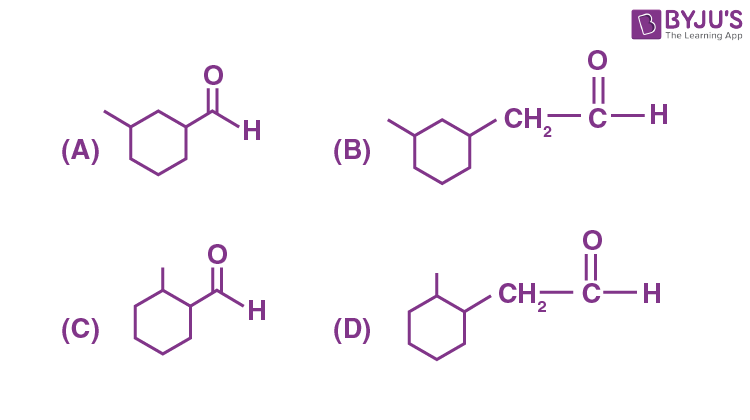Sol.γ-Methyl cyclohexane carbaldehyde

14. Compound ‘A’ undergoes following sequence of reactions to give compound ‘B’. The correct structure and chirality of compound ‘B’ is

[where Et is –C2H5]Compound ‘A’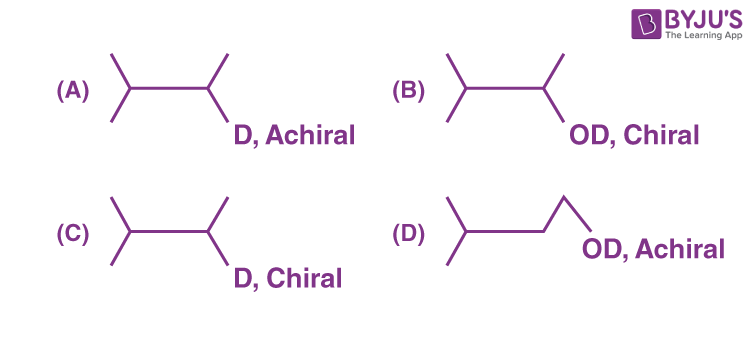Sol.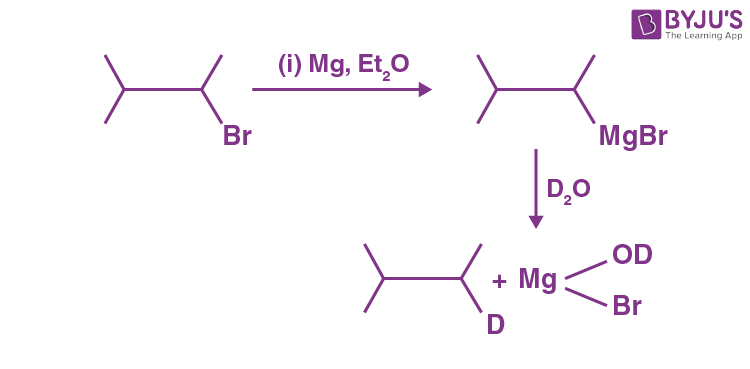15. Given below are two statements.

Statement I: The compound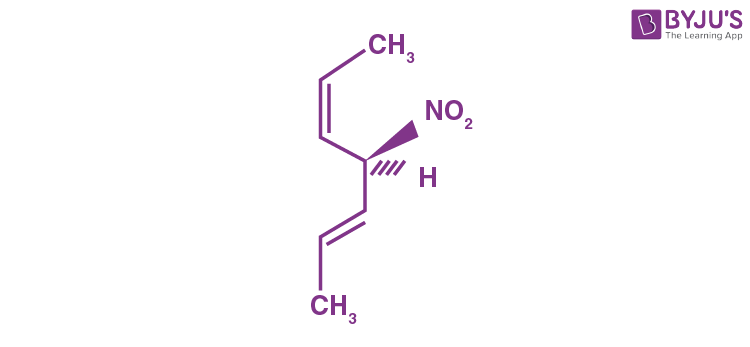(A) is optically active.

Statement II: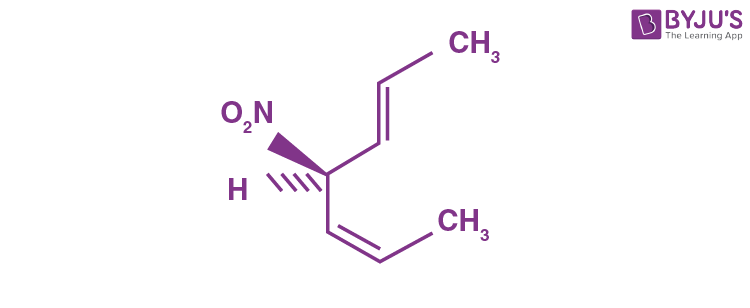is mirror image of above compound A.

In the light of the above statement, choose the most appropriate answer from the options given below.

(A) Both Statement I and Statement II are correct.

(B) Both Statement I and Statement II are incorrect.

(C) Statement I is correct but Statement II is incorrect.

(D) Statement I is incorrect but Statement II is correct.

Sol. Compound (A) in Statement-I and compound in Statement-II is not the mirror image of (I).

16. When ethanol is heated with conc. H2SO4, a gas is produced. The compound formed, when this gas is treated with cold dilute aqueous solution of Baeyer’s reagent, is

(A) Formaldehyde

(B) Formic acid

(C) Glycol

(D) Ethanoic acid

Sol.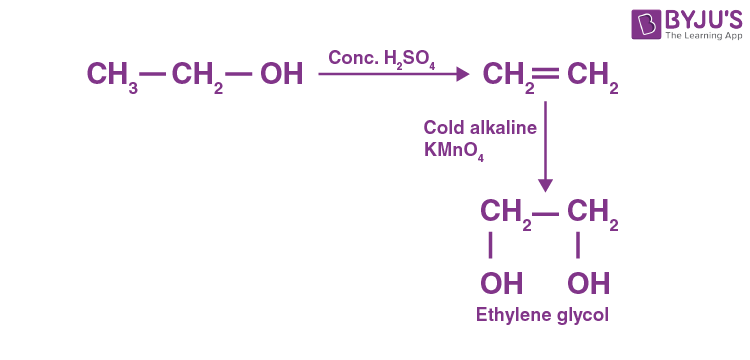17. The Hinsberg reagent is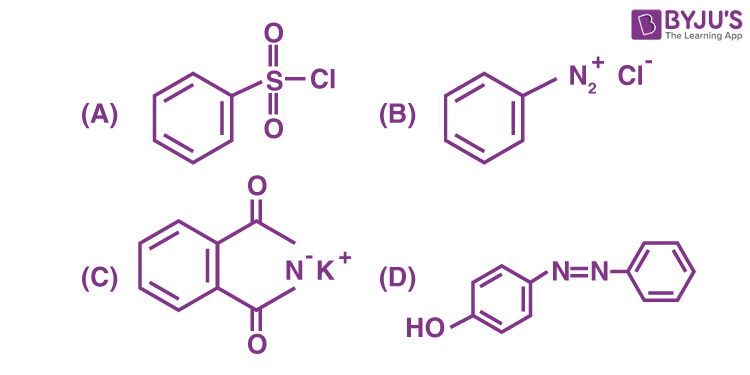Sol. Hinsberg reagent is :18. Which of the following is not a natural polymer?

(A) Protein

(B) Starch

(C) Rubber

(D) Rayon

Sol. Rayon is not natural polymer. It is semi-synthetic, rest all are natural polymers.

19. Given below are two statements. One is labelled as Assertion A and the other is labelled as Reason R.

Assertion A : Amylose is insoluble in water.

Reason R : Amylose is a long linear molecule with more than 200 glucose units.

In the light of the above statements, choose the correct answer from the options given below.

(A) Both A and R are correct and R is the correct explanation of A

(B) Both A and R are correct but R is NOT the correct explanation of A

(C) A is correct but R is not correct

(D) A is not correct but R is correct

Sol. Amylose is a linear polymer formed by combination of α-D glucose through 1, 4- glycosidic linkage.

It is water soluble.

So, assertion is incorrect.

20. A compound ‘X’ is a weak acid and it exhibits colour change at pH close to the equivalence point during neutralization of NaOH with CH3COOH. Compound ‘X’ exists in ionized from in basic medium. The compound ‘X’ is

(A) Methyl orange

(B) Methyl red

(C) Phenolphthalein

(D) Eriochrome Black T

Sol.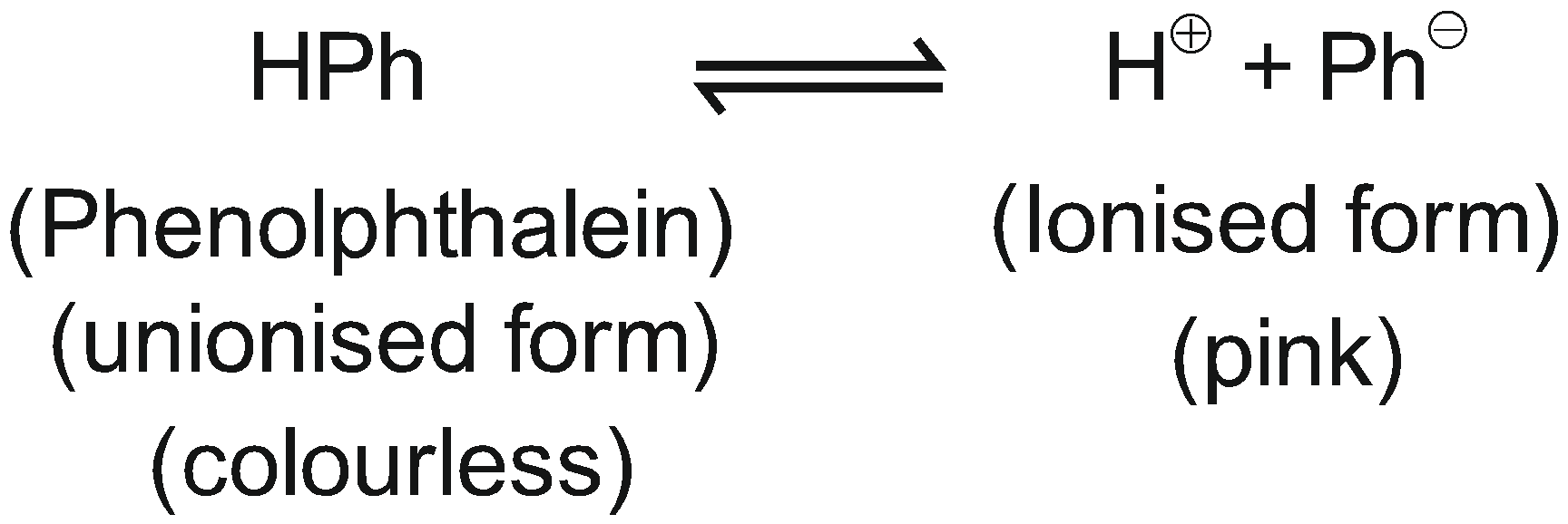In basic medium,

$$\begin{array}{l}[H^{\bigoplus}]\ \text{decreases}\end{array}$$
& therefore
$$\begin{array}{l}\text{more of}\ (Ph^\ominus)\ \text{is produced}.\end{array}$$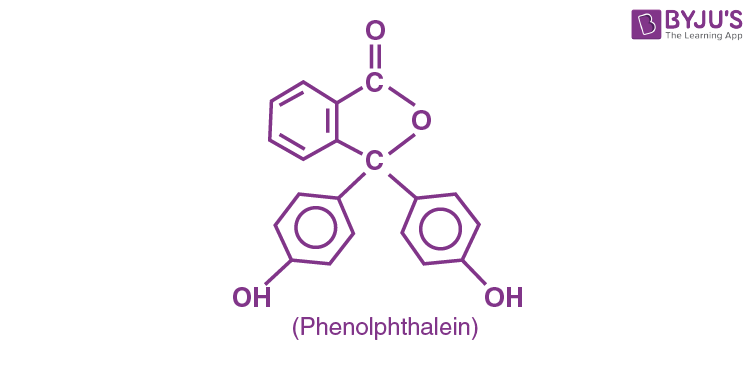SECTION – B

Numerical Value Type Questions: This section contains 10 questions. In Section B, attempt any five questions out of 10. The answer to each question is a NUMERICAL VALUE. For each question, enter the correct numerical value (in decimal notation, truncated/rounded-off to the second decimal place; e.g. 06.25, 07.00, –00.33, –00.30, 30.27, –27.30) using the mouse and the on-screen virtual numeric keypad in the place designated to enter the answer.

1. ‘x’ g of molecular oxygen (O2) is mixed with 200 g of neon (Ne). The total pressure of the non-reactive mixture of O2 and Ne in the cylinder is 25 bar. The partial pressure of Ne is 20 bar at the same temperature and volume. The value of ‘x’ is ___.

[Given : Molar mass of O2 = 32 g mol–1.

Molar mass of Ne = 20 g mol–1]

Sol.

$$\begin{array}{l}P_{O_2}= 25-20=5 \text{ bar}\end{array}$$
$$\begin{array}{l}P_{O_2}= X_{O_2} \times P_{\text{Total}}\end{array}$$
$$\begin{array}{l}\frac{5}{25}=\frac{n_{O_2}}{n_{O_2} + n_{Ne}}\end{array}$$
$$\begin{array}{l}\frac{1}{5}=\frac{x/32}{\frac{x}{32}+\frac{200}{20}}\Rightarrow \frac{x}{32}+10 = \frac{5x}{32}\end{array}$$
$$\begin{array}{l}\Rightarrow \frac{x}{8}=10 \\x = 80 \text{gm}\end{array}$$

2. Consider,

$$\begin{array}{l}PF_5, BrF_5, PCl_3, SF_6, \left[ICl_4\right]^–, ClF_3\ \text{and}\ IF_5.\end{array}$$

Amongst the above molecule(s)/ion(s), the number of molecule(s)/ion(s) having sp3d2 hybridisation is _____.

Sol.

$$\begin{array}{l}\begin{matrix}& & \text{Hybridisation of Central atom}\\ PF_5& \longrightarrow & sp^3d \\ BrF_5 & \longrightarrow & sp^3d^2 \\PCl_3 & \longrightarrow & sp^3 \\SF_6 & \longrightarrow & sp^3d^2 \\ICl_4^\ominus & \longrightarrow & sp^3d^2\\CIF_3 & \longrightarrow & sp^3d \\IF_5 & \longrightarrow & sp^3d^2\\\end{matrix}\end{array}$$

3. 1.80 g of solute A was dissolved in 62.5 cm3 of ethanol and freezing point of the solution was found to be 155.1 K. The molar mass of solute A is ____ g mol–1.

[Given : Freezing point of ethanol is 156.0 K.

Density of ethanol is 0.80 g cm–3.

Freezing point depression constant of ethanol is 2.00 K kg mol–1]

Sol.

$$\begin{array}{l}\Delta T_f = k_f m\end{array}$$
$$\begin{array}{l}0.9 = \frac{2 \times 1.8 \times 1000}{62.5 \times 0.8 \times M}\end{array}$$
$$\begin{array}{l}M= \frac{2 \times 1800}{62.5 \times 0.8 \times 0.9}\\= 80 ~\text{g/mol} \end{array}$$

4. For a cell,

$$\begin{array}{l}Cu\left(s\right) \left| Cu^{2+} \left(0.001M\right) || Ag^+ (0.01M) \right| Ag(s) \end{array}$$
the cell potential is found to be 0.43 V at 298 K. The magnitude of standard electrode potential for Cu2+/Cu is ______ × 10–2 V.

$$\begin{array}{l}\left[Given:E^\ominus_{Ag^+/Ag}=0.80 ~\text{V}~ \text{and}~ \dfrac{2.303RT}{F}=0.06~\text{V}\right]\end{array}$$

Sol.

$$\begin{array}{l}E=E^{\circ} – \frac{0.06}{2}\log\frac{[Cu^{2+}]}{[Ag^{\oplus}]^2}\end{array}$$
$$\begin{array}{l}=E^{\circ} – \frac{0.06}{2}\log \frac{0.001}{(0.01)^2}\end{array}$$
$$\begin{array}{l}0.43 = E^\circ – 0.03\\ E^\circ = 0.46 ~\text{V}\end{array}$$
$$\begin{array}{l}E_{Ag^\oplus/Ag}^\circ-E_{cu^{2+}/Cu}^\circ = 0.46\end{array}$$
$$\begin{array}{l}\therefore E_{cu^{2+}/Cu}^\circ = 0.8 – 0.46\end{array}$$

= 0.34 V

= 34 × 10-2 V

5. Assuming 1μg of trace radioactive element X with a half life of 30 years is absorbed by a growing tree. The amount of X remaining in the tree after 100 years is ______ × 10–1 μg.

[Given : ln 10 = 2.303; log 2 = 0.30]

Sol.

$$\begin{array}{l}kt = ln \dfrac{1}{1-X}\end{array}$$
$$\begin{array}{l}\frac{0.693}{30}(100)=\ln \frac{1}{1-X}\end{array}$$
$$\begin{array}{l}2.303 = 2.303 \log \frac{1}{1-X}\Rightarrow \frac{1}{1-X} = 10\end{array}$$
$$\begin{array}{l}\Rightarrow 1 = 10 – 10X\end{array}$$
$$\begin{array}{l}\Rightarrow X = \frac{9}{10}= 0.9 ~\mu \text{g}\end{array}$$
$$\begin{array}{l}\text{Amount of X remaining} = 1 – X\\ = 1 – 0.9 = 0.1 \mu \text{g}\\ = 1 \times 10^{-1} \mu \text{g}\end{array}$$

6. Sum of oxidation state (magnitude) and coordination number of cobalt in Na[Co(bpy)Cl4] is____.

(Given : bpy =)

Sol. Na [Co(bpy)Cl4]

Oxidation state of cobalt = + 3

Coordination number of cobalt = 6

[As bpy is bidentate]

So, sum = 9

7. Consider the following sulphur based oxoacids.

$$\begin{array}{l}H_2SO_3, H_2SO_4, H_2S_2O_8 ~\text{and}~ H_2S_2O_7.\end{array}$$

Amongst these oxoacids, the number of those with peroxo (O–O) bonds is_____.

Sol.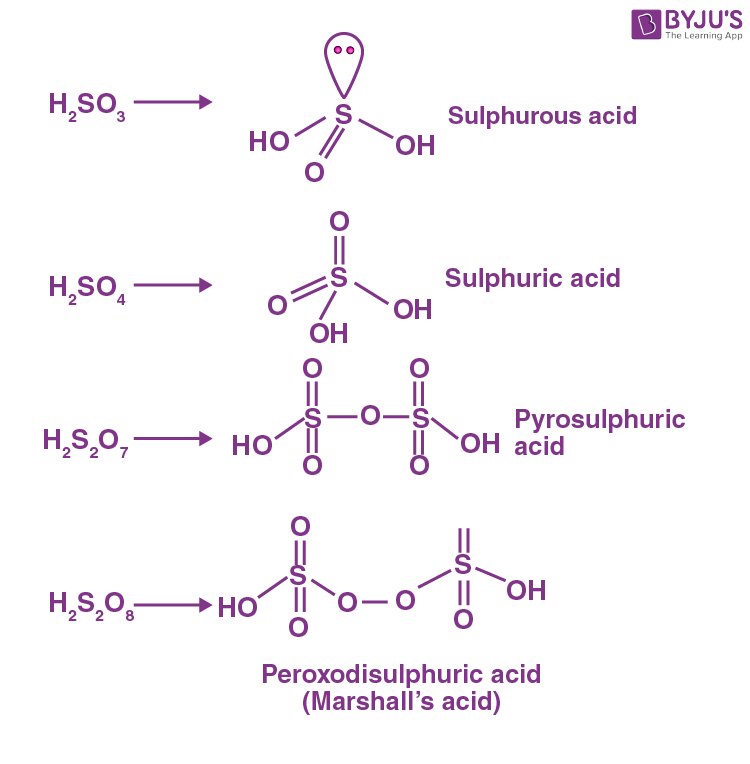8. A 1.84 mg sample of polyhydric alcoholic compound ‘X’ of molar mass 92.0 g/mol gave 1.344 mL of H2 gas at STP. The number of alcoholic hydrogens present in compound ‘X’ is____.

Sol.

$$\begin{array}{l}\text{Moles of H2 produced at STP} \\ =\dfrac{1.344 \times 10^{-3}}{22.4}\\ = 6 \times 10^{-5} \text{mole}\end{array}$$
$$\begin{array}{l} \therefore \text{Moles of hydrogen atom produced}\\ = 12 \times 10^{-5}~\text{mol} \end{array}$$
$$\begin{array}{l} \text{Moles of organic compound}\\ =\dfrac{1.84 \times 10^{-3}}{92}\\ = 2 \times 10^{-5} \end{array}$$
$$\begin{array}{l} \therefore \text{Number of alcoholic hydrogen present}\\=\frac{12 \times 10^{-5}}{2 \times 10^{-5}}=6\end{array}$$

9. The number of stereoisomers formed in a reaction of (±) Ph (C = O) C (OH) (CN) Ph with HCN is_____.

[where Ph is –C6H5]

Sol.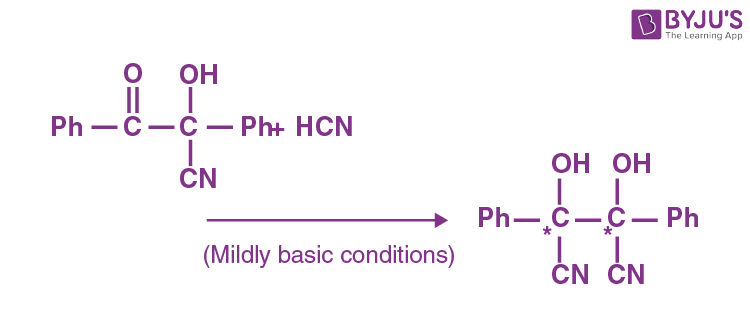Number of stereoisomers = 3

10. The number of chlorine atoms in bithionol is______.

Sol. Number of chlorine atoms in bithionol = 4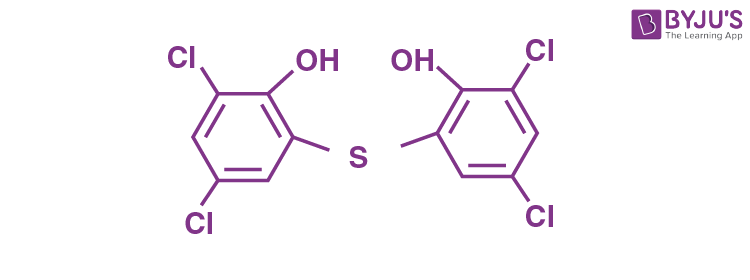### Download PDF of JEE Main 2022 July 29 Shift 2 Chemistry Paper & Solutions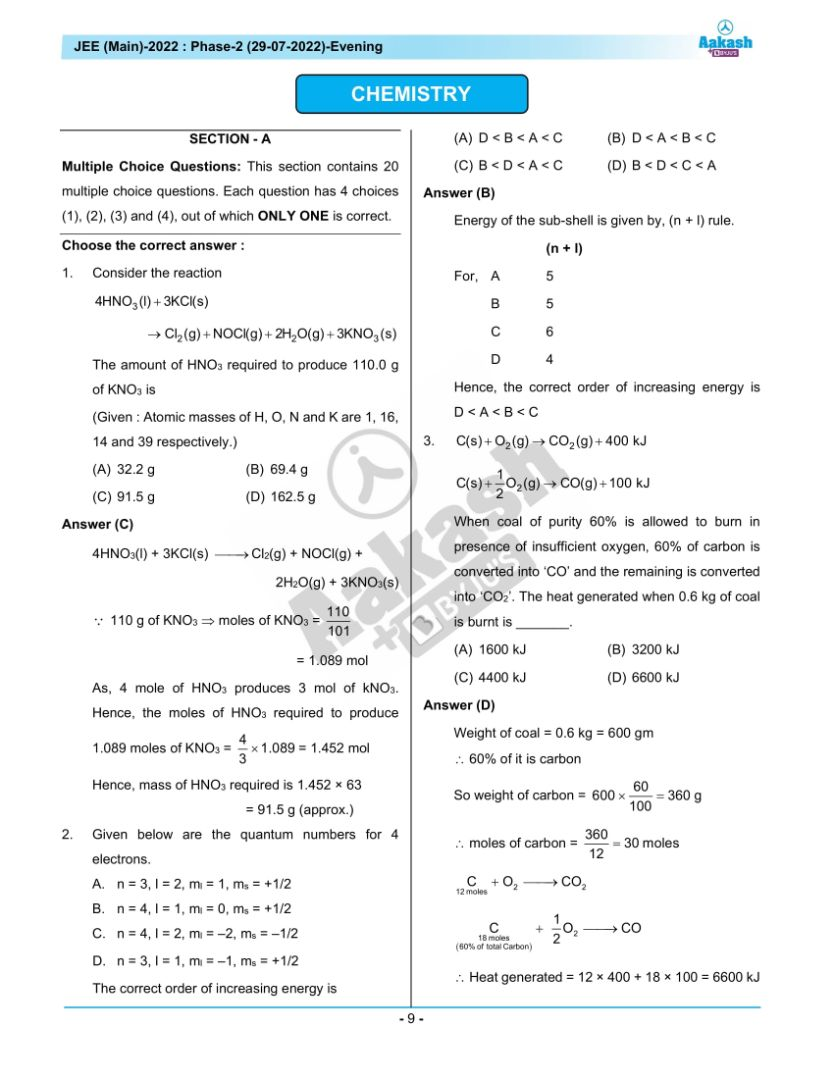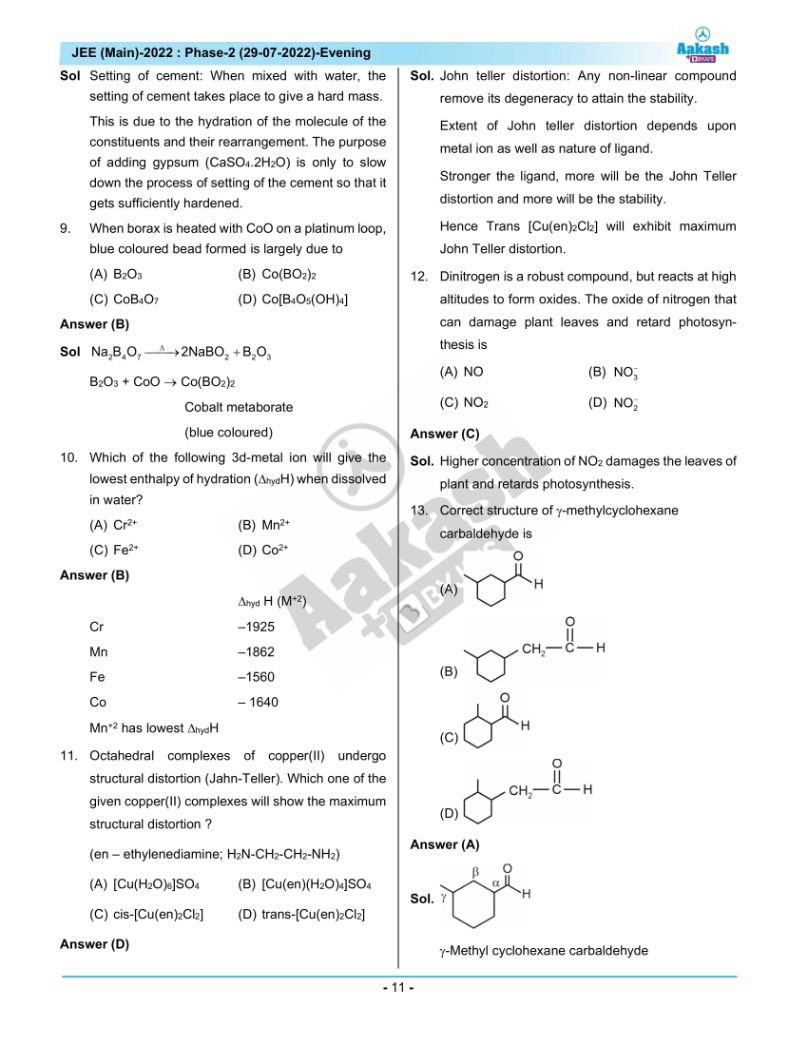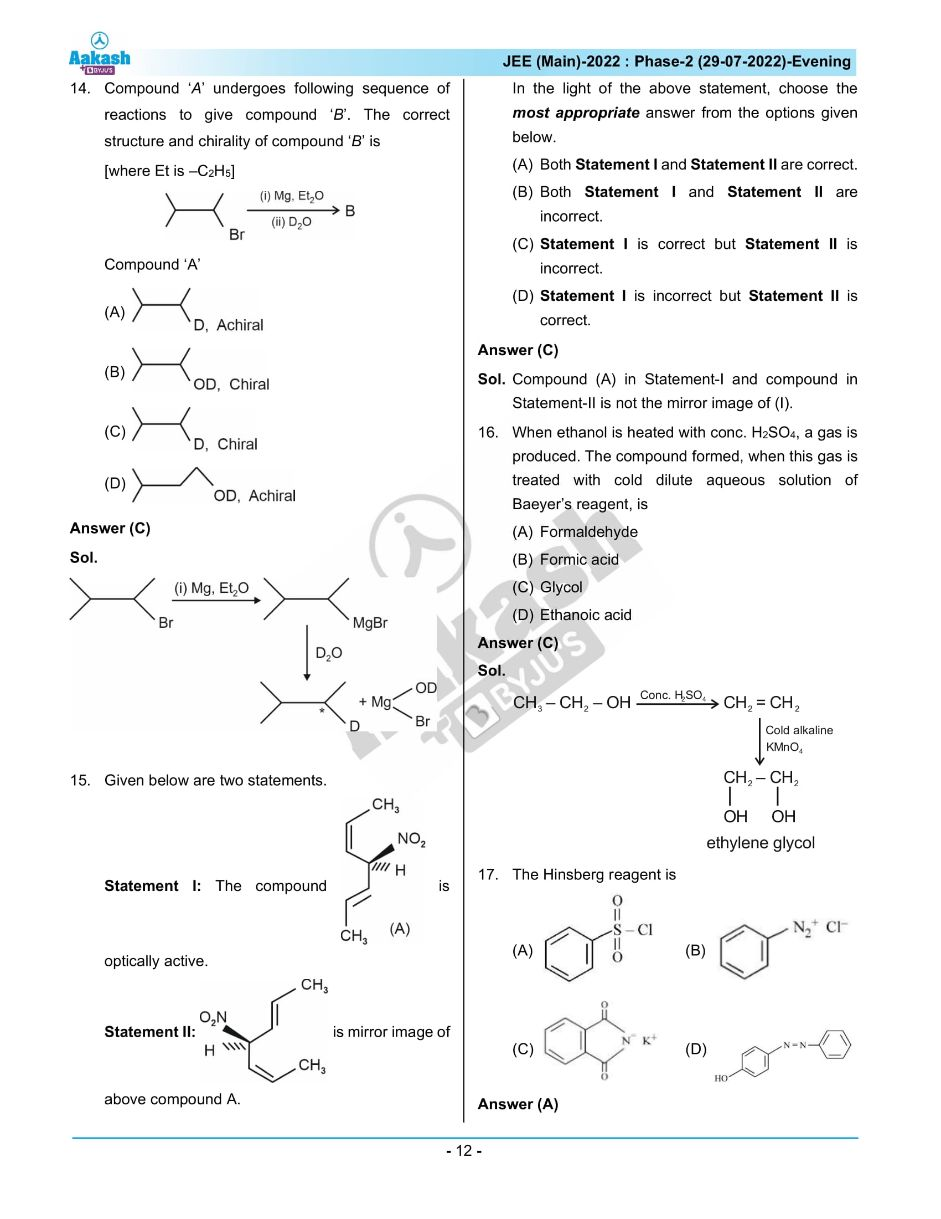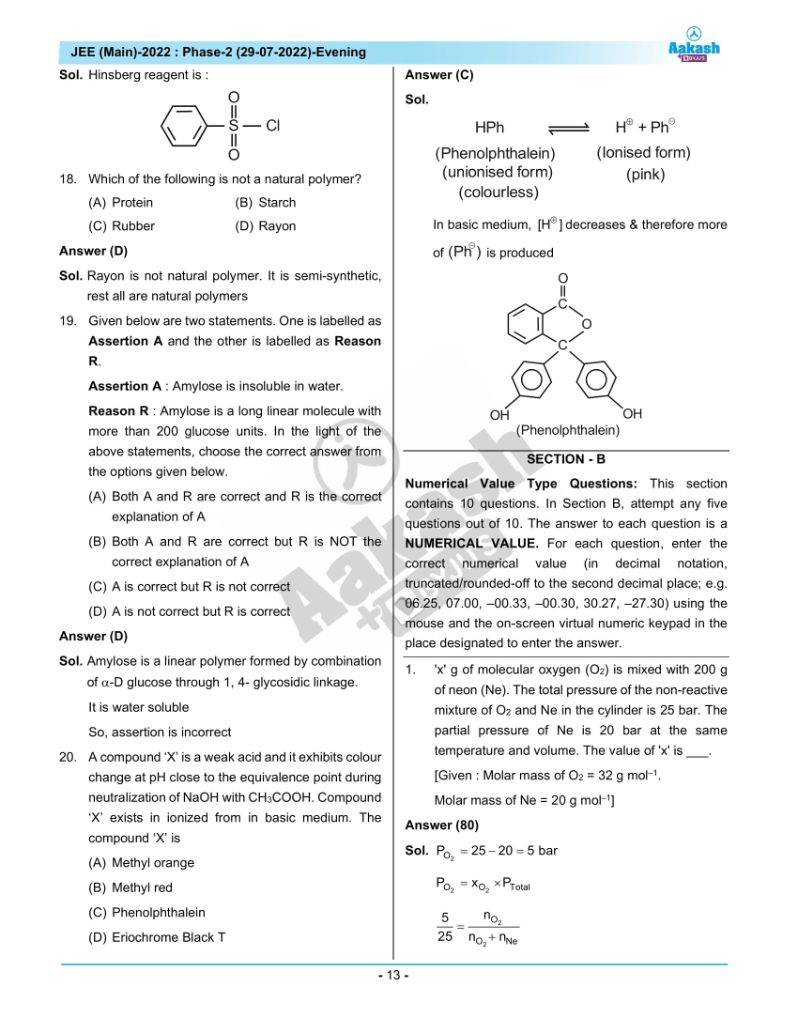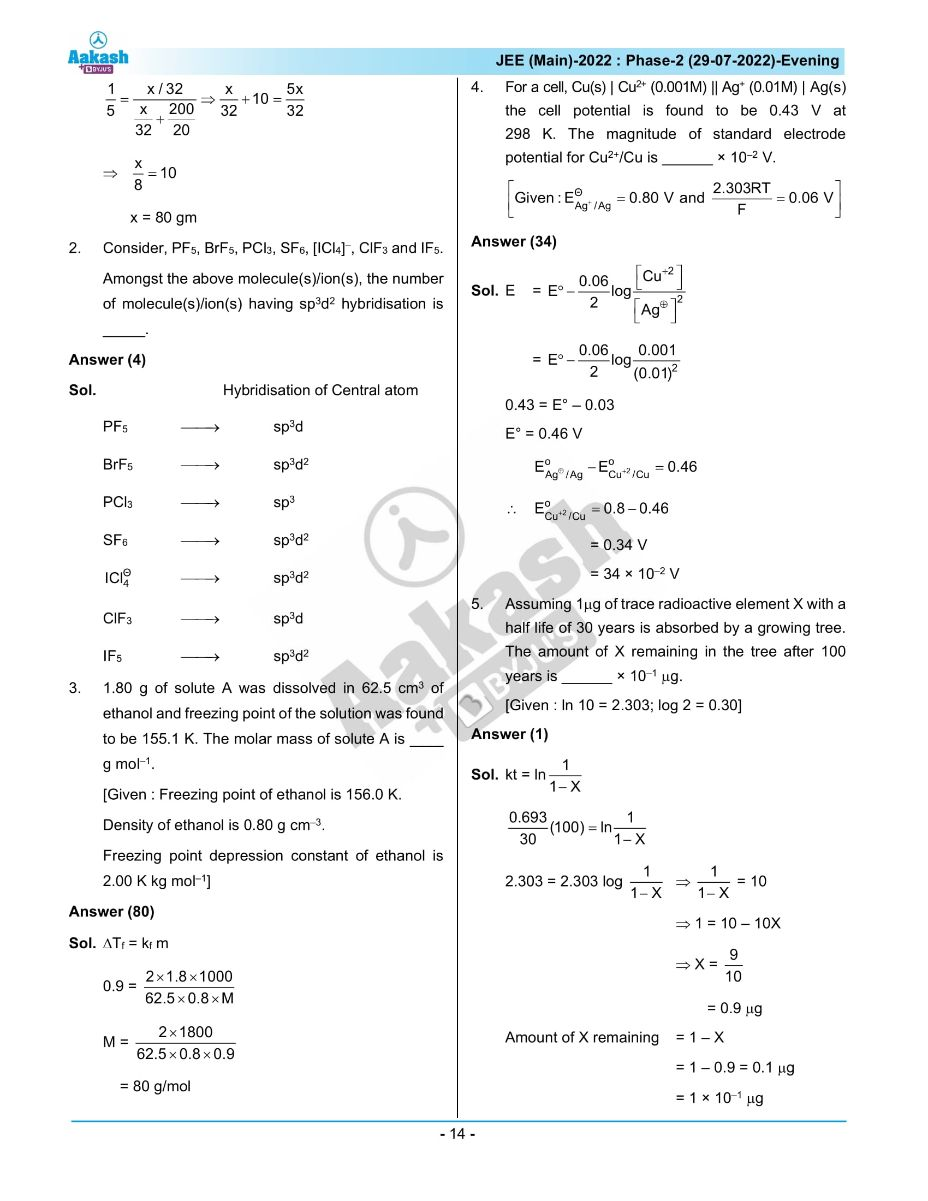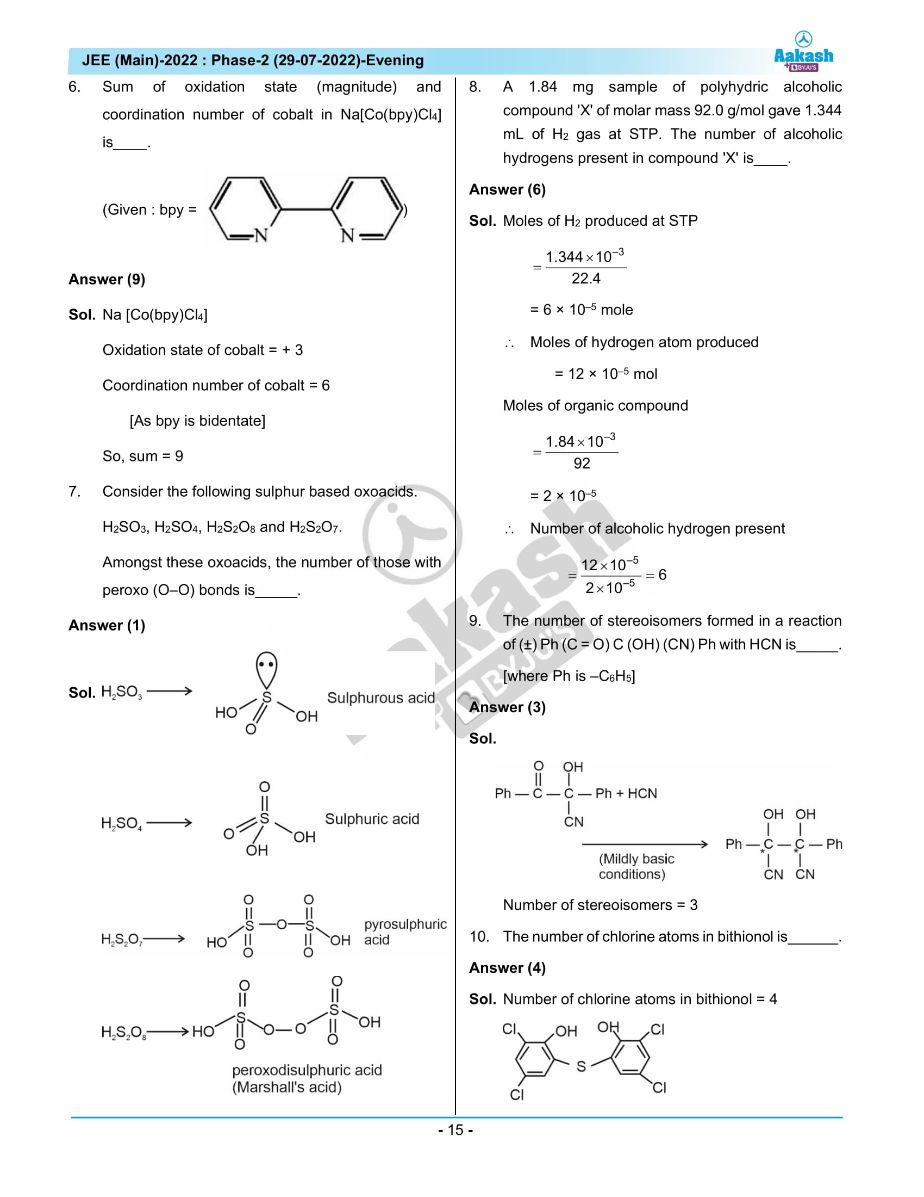## JEE Main 2022 July 29th Shift 2 Paper Analysis